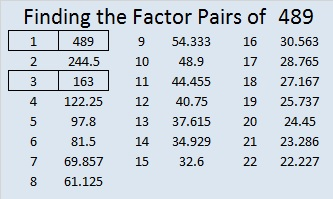# 489 and Level 2

489 is the ninth octahedral number.  Its square root starts out in quite a memorable way: √489 ≈ 22.113344, but after those six decimal places, it looks as irrational as can be.

Print the puzzles or type the solution on this excel file: 10 Factors 2015-05-11

—————————————————————————————————

• 489 is a composite number.
• Prime factorization: 489 = 3 x 163
• The exponents in the prime factorization are 1 and 1. Adding one to each and multiplying we get (1 + 1)(1 + 1) = 2 x 2 = 4. Therefore 489 has exactly 4 factors.
• Factors of 489: 1, 3, 163, 489
• Factor pairs: 489 = 1 x 489 or 3 x 163
• 489 has no square factors that allow its square root to be simplified. √489 ≈ 22.113344—————————————————————————————————